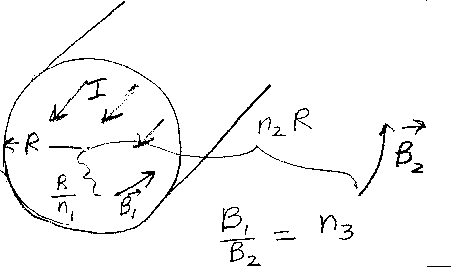Problem C3: The figure above shows a cylindrical wire of radius R. The wire is infinitly long and has a total current I. The current I is uniformly distributed in the wire. What is the ratio of the magnetic field B1 at the point r1=R/n1 to the magnetic field B2 at the point r2=n2R? That is, if B1/B2 = n3, what is n3?n1 = n2 = Input n3:
If you are currently in my class, you can record your grade by entering your name and student ID number (without the leading zeros) below and clicking on "record grade".
 First Name = Last Name = ID = Problem: